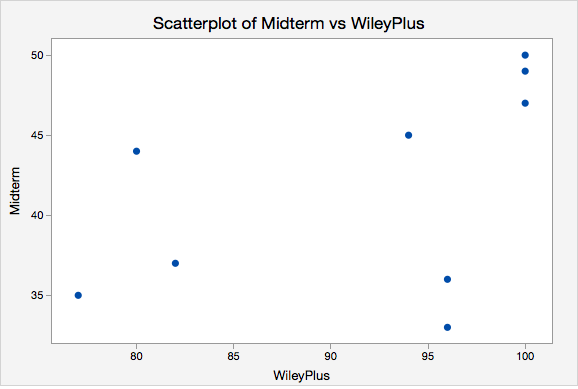# 3.4.2.2 - Example of Computing r by Hand (Optional)

Again, you will not need to compute $$r$$ by hand in this course. This example is meant to show you how $$r$$ is computed with the intention of enhancing your understanding of its meaning. In this course, you will always be using Minitab Express or StatKey to compute correlations.

In this example we have data from a random sample of $$n = 9$$ World Campus STAT 200 students from the Spring 2017 semester. WileyPlus scores had a maximum possible value of 100. Midterm exam scores had a maximum possible value of 50. Remember, the $$x$$ and $$y$$ variables do not need to be on the same metric to compute a correlation.

ID WileyPlus Midterm
A 82 37
B 100 47
C 96 33
D 96 36
E 80 44
F 77 35
G 100 50
H 100 49
I 94 45

Minitab Express was used to construct a scatterplot of these two variables. We need to examine the shape of the relationship before determining if Pearson's $$r$$ is the appropriate correlation coefficient to use. Pearson's $$r$$ can only be used to check for a linear relationship. For this example I am going to call WileyPlus grades the $$x$$ variable and midterm exam grades the $$y$$ variable because students completed WileyPlus assignments before the midterm exam.### Summary Statistics

From this scatterplot we can determine that the relationship may be weak, but that it is reasonable to consider a linear relationship. If we were to draw a line of best fit through this scatterplot we would draw a straight line with a slight upward slope. Now, we'll compute Pearson's $$r$$ using the $$z$$ score formula. The first step is to convert every WileyPlus score to a $$z$$ score and every midterm score to a $$z$$ score. When we constructed the scatterplot in Minitab Express we were also provided with summary statistics including the mean and standard deviation for each variable which we need to compute the $$z$$ scores.

Statistics
Variable N* Mean StDev Minimum Maximum
Midterm 9 41.778 6.534 33.000 50.000
WileyPlus 9 91.667 9.327 77.000 100.000
ID WileyPlus $$z_x$$
A 82 $$\frac{82-91.667}{9.327}=-1.036$$
B 100 $$\frac{100-91.667}{9.327}=0.893$$
C 96 $$\frac{96-91.667}{9.327}=0.465$$
D 96 $$\frac{96-91.667}{9.327}=0.465$$
E 80 $$\frac{80-91.667}{9.327}=-1.251$$
F 77 $$\frac{77-91.667}{9.327}=-1.573$$
G 100 $$\frac{100-91.667}{9.327}=0.893$$
H 100 $$\frac{100-91.667}{9.327}=0.893$$
I 94 $$\frac{94-91.667}{9.327}=0.250$$
z-score
$$z_x=\frac{x - \overline{x}}{s_x}$$

A positive value in the $$z_x$$ column means that the student's WileyPlus score is above the mean. Now, we'll do the same for midterm exam scores.

ID Midterm $$z_y$$
A 37 $$\frac{37-41.778}{6.534}=-0.731$$
B 47 $$\frac{47-41.778}{6.534}=0.799$$
C 33 $$\frac{33-41.778}{6.534}=-1.343$$
D 36 $$\frac{36-41.778}{6.534}=-0.884$$
E 44 $$\frac{44-41.778}{6.534}=0.340$$
F 35 $$\frac{35-41.778}{6.534}=-1.037$$
G 50 $$\frac{50-41.778}{6.534}=1.258$$
H 49 $$\frac{49-41.778}{6.534}=1.105$$
I 45 $$\frac{45-41.778}{6.534}=0.493$$

Our next step is to multiply each student's WileyPlus $$z$$ score with his or her midterm exam score.

ID $$z_x$$ $$z_y$$ $$z_x z_y$$
A -1.036 -0.731 0.758
B 0.893 0.799 0.714
C 0.465 -1.343 -0.624
D 0.465 -0.884 -0.411
E -1.251 0.340 -0.425
F -1.573 -1.037 1.631
G 0.893 1.258 1.124
H 0.893 1.105 0.988
I 0.250 0.493 0.123

A positive "cross product" (i.e., $$z_x z_y$$) means that the student's WileyPlus and midterm score were both either above or below the mean. A negative cross product means that they scored above the mean on one measure and below the mean on the other measure. If there is no relationship between $$x$$ and $$y$$ then there would be an even mix of positive and negative cross products; when added up these would equal around zero signifying no relationship. If there is a relationship between $$x$$ and $$y$$ then these cross products would primarily be going in the same direction. If the correlation is positive then these cross products would primarily be positive. If the correlation is negative then these cross products would primarily be negative; in other words, students with higher $$x$$ values would have lower $$y$$ values and vice versa. Let's add the cross products here and compute our $$r$$ statistic.

$$\sum z_x z_y = 0.758+0.714-0.624-0.411-0.425+1.631+1.124+0.988+0.123=3.878$$

$$r=\frac{3.878}{9-1}=0.485$$

There is a positive, moderately strong, relationship between WileyPlus scores and midterm exam scores in this sample.